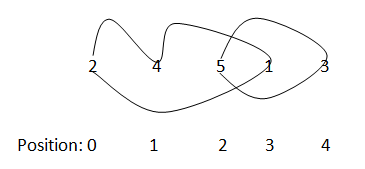# Minimum swaps required to sort an array in Java

Here, we will learn to get/find the minimum swaps that are required to sort an array using java program.
Submitted by Anamika Gupta, on August 08, 2018

Problem:

In this problem, we would have an unordered array with consecutive distinct natural numbers [1,2,3,..n], where n is the size of the array. We have to find the minimum number of swaps required to sort the array in ascending order.

Note: Think and try by yourself before looking to the solution...

Solution:

This problem can be solved easily by observing the actual position of elements and their current position , the actual position of element in sorted array will be the a[cur]-1 (element-1), by tracking the actual position of element if we come back to the current element then there exist a cycle , then count the size of that cycle , the number of swaps will be cycling size-1, do this for all the cycles and add them together.

Example:

Let an array: A =[2, 4, 5, 1, 3]There exist two cycles:
Cycle 1: 2 → 4 → 1 → 2
Cycle 2: 5 → 3 → 5

Size of cycle = number of nodes:
Size of cycle 1=3
Size of cycle 2=2

Number of swaps: (3-1)+(2-1) = 3

Program:

```import java.io.*;
import java.math.*;
import java.util.*;

public class Swap {
static int minimumSwaps(int[] arr) {
int swap=0;
boolean visited[]=new boolean[arr.length];

for(int i=0;i<arr.length;i++){
int j=i,cycle=0;

while(!visited[j]){
visited[j]=true;
j=arr[j]-1;
cycle++;
}

if(cycle!=0)
swap+=cycle-1;
}
return swap;
}

public static void main(String[] args) {

Scanner scanner = new Scanner(System.in);
int n = scanner.nextInt();
int[] arr = new int[n];

for (int i = 0; i < n; i++) {
arr[i] = scanner.nextInt();
}

int res = minimumSwaps(arr);
System.out.println(res);
scanner.close();
}
}
```

Output

```    4
4 3 2 1
2
```

Top MCQs

Languages: » C » C++ » C++ STL » Java » Data Structure » C#.Net » Android » Kotlin » SQL
Web Technologies: » PHP » Python » JavaScript » CSS » Ajax » Node.js » Web programming/HTML
Solved programs: » C » C++ » DS » Java » C#
Aptitude que. & ans.: » C » C++ » Java » DBMS
Interview que. & ans.: » C » Embedded C » Java » SEO » HR
CS Subjects: » CS Basics » O.S. » Networks » DBMS » Embedded Systems » Cloud Computing
» Machine learning » CS Organizations » Linux » DOS
More: » Articles » Puzzles » News/Updates